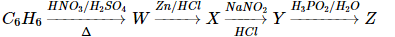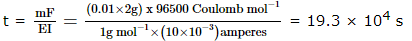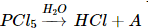# WBJEE Chemistry Test - 12

## 40 Questions MCQ Test WBJEE Sample Papers, Section Wise & Full Mock Tests | WBJEE Chemistry Test - 12

Description
Attempt WBJEE Chemistry Test - 12 | 40 questions in 60 minutes | Mock test for JEE preparation | Free important questions MCQ to study WBJEE Sample Papers, Section Wise & Full Mock Tests for JEE Exam | Download free PDF with solutions
QUESTION: 1

Solution:
QUESTION: 2

Solution:
QUESTION: 3

### Scurvy is caused due to deficiency of

Solution:
QUESTION: 4
In Rosenmund reaction,
Solution:
QUESTION: 5
Which of the following is soluble in yellow ammonium sulphide?
Solution:
QUESTION: 6
The phenomenon in which white transparent crystal changes into white powder is called
Solution:
QUESTION: 7
What is the wave number of 4th line in Balmer series of Hydrogen spectrum? (R = 1,09,677cm-1)
Solution:
QUESTION: 8

Which of the following does not have sp2-hybridised carbon?

Solution:
QUESTION: 9
Which pair of elements among the following will form most stable ionic bond
Solution:
QUESTION: 10
Which among the following is not a state function ?
Solution:
QUESTION: 11
A vessel at equilibrium, contains SO₃, SO₂ and O₂. Now some helium gas is added, so that total pressure increases while temperature and volume remain constant. According to Le-Chatelier's principle, the dissociation of SO₃
Solution:
QUESTION: 12
A first order reaction is 10% complete in 20 min. The time taken for 19% completion is
Solution:
QUESTION: 13
The systematic name of (CH₃)₂CH-COOH is
Solution:
QUESTION: 14
Diazo coupling is useful to prepare some
Solution:
QUESTION: 15

Identify the reaction for which Δ H ≠ Δ E

Solution:
QUESTION: 16
Which among the following is the strongest base?
Solution:
QUESTION: 17

The chemical formula of Iron (III) hexacyanoferrate (II) is

Solution:
QUESTION: 18

0.24 g of a volatile gas upon vapourisation gives 45 ml vapour of N.T.P. what will be vapour density of the substance ? (Density of H₂ = 0.089 gl-1)

Solution:
QUESTION: 19

Which one of the following is correct about stability of the given ions?

Solution:
QUESTION: 20
When bauxite powder is mixed with coke and reacted with nitrogen at 2075K, carbon compound and X are formed. What is the gas formed when X is reacted with water
Solution:
QUESTION: 21
Which of the following substances is used as fertilizer ?
Solution:
QUESTION: 22
Reaction of potassium with water is
Solution:
QUESTION: 23
The number of π-bonds in CH₂ = CH-CH = CH-C ≡ CH is
Solution:
QUESTION: 24
The half-life of a radioactive isotope is 2.3 hours. Its disintegration constant will be
Solution:
QUESTION: 25
Which of the following is plasticizer
Solution:
QUESTION: 26
Aniline in a set of reactions yielded a product D

The structure of D is
Solution:
QUESTION: 27
Buna-N is prepared by the polymerisation of
Solution:
QUESTION: 28
In which of the following reaction there is no change in oxidation number
Solution:
QUESTION: 29

The second order Bragg diffraction of X-rays with λ = 1.00 Å from a set of parallel planes in a metal occurs at an angle of 600. The distance between the scattering planes in the crystal is

Solution:
QUESTION: 30
Consider the following statements about chirality :
1. Molecules which are not superimposable on their mirror images are achiral
2. A chiral molecule can have simple axis of symmetry
3. A carbon atom of which four different groups are attached is a chiral centre
4. A compound whose molecules are achiral exhibits optical activity
Which of the statements given above are correct ?
Solution:
QUESTION: 31

Solubility product constants (K(sp)) of salts of types MX, MX2 and M3X at temperature 'T' are 4.0 x 10-8, 3.2 x 10-14 and 2.7 x 10-15, respectively. Solubilities (mol dm-3) of the salts at temperature 'T' are in the order

Solution:
QUESTION: 32

'Z' in the following sequence of reactions isSolution:
QUESTION: 33

Electrolysis of dilute aqueous NaCl solution was carried out by passing 10 milli ampere current. The time required to liberate 0.01 mol of H2 gas at the cathode is (1 Faraday = 96500 C mol-1)

Solution:

According to Faraday's first law of electrolysis:
m = EIt/F
Where:
m = mass of substance
E = equivalent weight of substance
I = current in amperes
t = time required
F = 96,500 Coulombs
t = mF EI = (0.01x 2g) x 96500 CoulombQUESTION: 34

In the following reactionthe product 'A' is

Solution:
QUESTION: 35

Both [Ni(CO)4] and [Ni(CN)4](2-) are diamagnetic. The hybridisations of nickel in these complexes, respectively, are

Solution:
QUESTION: 36

Optical activity is expected for :

Solution:
QUESTION: 37

Chlorine atom does not differ from chloride ion in the number of which of the following?

Solution:
QUESTION: 38

Which of the following statements is/are true about an azeotropic mixture?

Solution:
QUESTION: 39

If x and y are arbitrary extensive variables, then

Solution:
QUESTION: 40

If Pand Ps are the V.P. of solvent and its solution respectively and N1 and N2 are the moles of solute and solvent then

Solution:Use Code STAYHOME200 and get INR 200 additional OFF Use Coupon Code